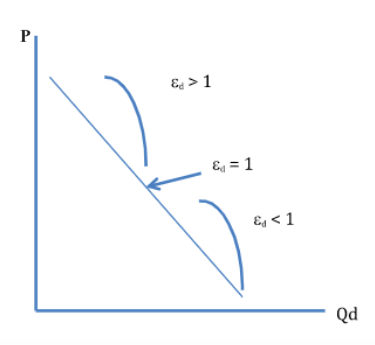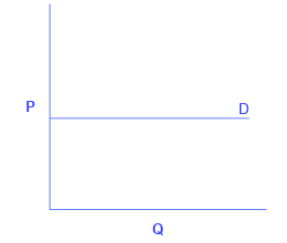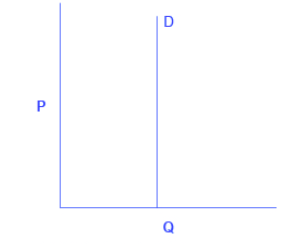## Categories of Elasticity

### Learning Objectives

• Explain and compare the graphs for the following types of elasticities: elastic, inelastic, unitary, infinite, and zero

The language of elasticity can sometimes be confusing. We use the word elasticity to describe the property of responsiveness in economic variables. We also describe the responsiveness as (relatively) elastic or (relatively) inelastic. It gets worse. We can also describe elasticity as perfectly elastic or perfectly inelastic. How to we keep these different meanings understood? That is the purpose of this section.

We mentioned previously that elasticity measurements are divided into three main ranges: elastic, inelastic, and unitary, corresponding to different parts of a linear demand curve.

Demand is described as elastic when the computed elasticity is greater than 1, indicating a high responsiveness to changes in price. Computed elasticities that are less than 1 indicate low responsiveness to price changes and are described as inelastic demand. Unitary elasticities indicate proportional responsiveness of  demand. In other words, the percent change in quantity demanded is equal to the percent change in price, so the elasticity equals 1. These ranges are summarized in Table 1, below.

Table 1. Three Categories of Elasticity: Elastic, Inelastic, and Unitary
If . . . Then . . . And It’s Called . . .
% change in quantity > % change in price Computed Elasticity > 1 Elastic
% change in quantity = % change in price Computed Elasticity = 1 Unitary
% change in quantity < % change in price Computed Elasticity < 1 Inelastic

It is important to note that both elastic and inelastic are relative terms, as shown in Figure 1, below. As one moves down the demand curve from top left to bottom right, the measured elasticity is much greater than one (very elastic), then just greater than one (somewhat elastic), then equal to one (unitary elastic, then less than one (somewhat inelastic), and finally much less than one (very inelastic). Note that the epsilon symbol, ε, is often used to represent elasticity.Figure 1. Variations in Elasticity. As you saw earlier, price elasticity of demand ranges from more than 1 at high prices and less than 1 at low prices. Measured elasticities decreases as one moves down the demand curve from left to right.

## Polar Cases of Elasticity

There are also two extreme cases of elasticity: when computed elasticity equals zero and when it’s infinite. We will describe each case.

A perfectly (or infinitely) elastic demand curve refers to the extreme case in which the quantity demanded (Qd) increases by an infinite amount in response to any decrease in price at all. Similarly, quantity demanded drops to zero for any increase in the price. A perfectly elastic demand curve is horizontal, as shown in Figure 2, below. While it’s difficult to think of real world example of infinite elasticity, it will be important when we study perfectly competitive markets. It’s a situation where consumers are extremely sensitive to changes in price. Say, for example, if the price of cruises to the Caribbean decreased, everyone would buy tickets (i.e., quantity demanded would increase to infinity), or when the price of cruises to the Caribbean increased, not a single person would be on the boat (i.e., quantity demanded would decrease to zero). Perfectly elastic demand is an “all or nothing” thing!Figure 2. Infinite Elasticity. This shows a perfectly elastic demand curve.  The horizontal line shows that an infinite quantity will be demanded at a specific price. The quantity demanded is extremely responsive to price changes, moving from zero for prices close to P to infinite when prices reach P.

While perfectly inelastic demand is an extreme case, necessities with no close substitutes are likely to have highly inelastic demand curves. This is the case with life-saving prescription drugs, for example. Consider a person with kidney failure who needs insulin to stay alive. A specific quantity of insulin is prescribed to the patient. If the price of insulin decreases, the patient can’t stock up and save it for the future. If the price of insulin increases, the patient will continue to purchase the same quantity needed to stay alive. Perfectly inelastic demand means that quantity demanded remains the same when price increases or decreases. Consumers are completely unresponsive to changes in price.Figure 3. Zero Elasticity. A perfectly inelastic demand curve. The vertical line shows that at any price, the quantity demanded remains the same. The measured elasticity is zero.

Final note: even though perfectly elastic and perfectly inelastic curves correspond to horizontal and vertical curves, remember that, in general, elasticity is not the same as the slope.

### Watch It

Watch this video to see graphed examples of perfectly inelastic, relatively inelastic, unit elastic, relatively elastic, and perfectly elastic demand.

### Try It

These questions allow you to get as much practice as you need, as you can click the link at the top of the first question (“Try another version of these questions”) to get a new set of questions. Practice until you feel comfortable doing the questions.

### Glossary

perfectly (or infinitely) elastic:
the extremely elastic situation of demand or supply where quantity changes by an infinite amount in response to any change in price; horizontal in appearance
perfectly inelastic:
the highly inelastic case of demand in which a percentage change in price, no matter how large, results in zero change in the quantity; thus, the price elasticity of demand is zero; vertical in appearance
(relatively) elastic:
the percentage change in quantity demanded is greater than the percentage change in price; measured price elasticity of demand is greater than one (in absolute value)
(relatively) inelastic:
the percentage change in quantity demanded is less than the percentage change in price; measure price elasticity of demand is less than one (in absolute value)
unitary elastic:
when a given percent price change in price leads to an equal percentage change in quantity demanded

## Contribute!

Did you have an idea for improving this content? We’d love your input.